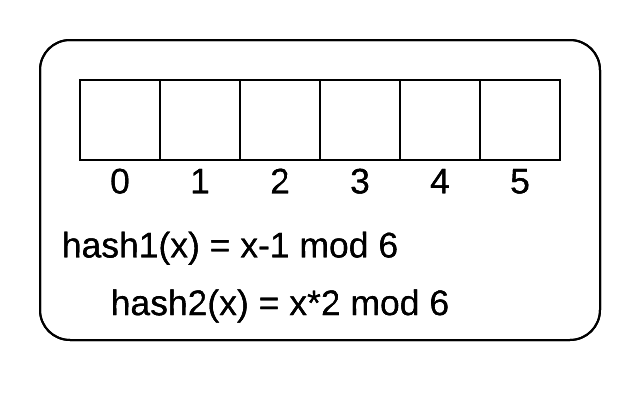What will happen to the cuckoo hash table?

The following image shows an empty cuckoo filter. There are 6 buckets and 2 hash functions. The hash functions are shown. The following 5 numbers are inserted into the hash table.

[3, 7, 14, 15, 19]Cukoo filter

For reference, here is how the cuckoo filter works:

 1 2 3 4 5 6 7 8 9 10 11 12 13 14 15 16 17 Parameters of the Filter: 1. Two Hash Functions: h1 and h2 2. An array B with n buckets. The i-th bucket will be called B[i] Input: L, a list of elements to be inserted into the cuckoo filter. Algorithm: While L is not empty: Let x be the first item in the list L. Remove x from the list. If B[h1(x)] is empty: place x in B[h1(x)] Else, If B[h2(x) is empty]: place x in B[h2(x)] Else: Let y be the element in B[h2(x)]. Prepend y to L place x in B[h2(x)]

Fill your answer in as a list of numbers. Leave a list slot empty if the corresponding bucket in the cuckoo table stays empty.

×